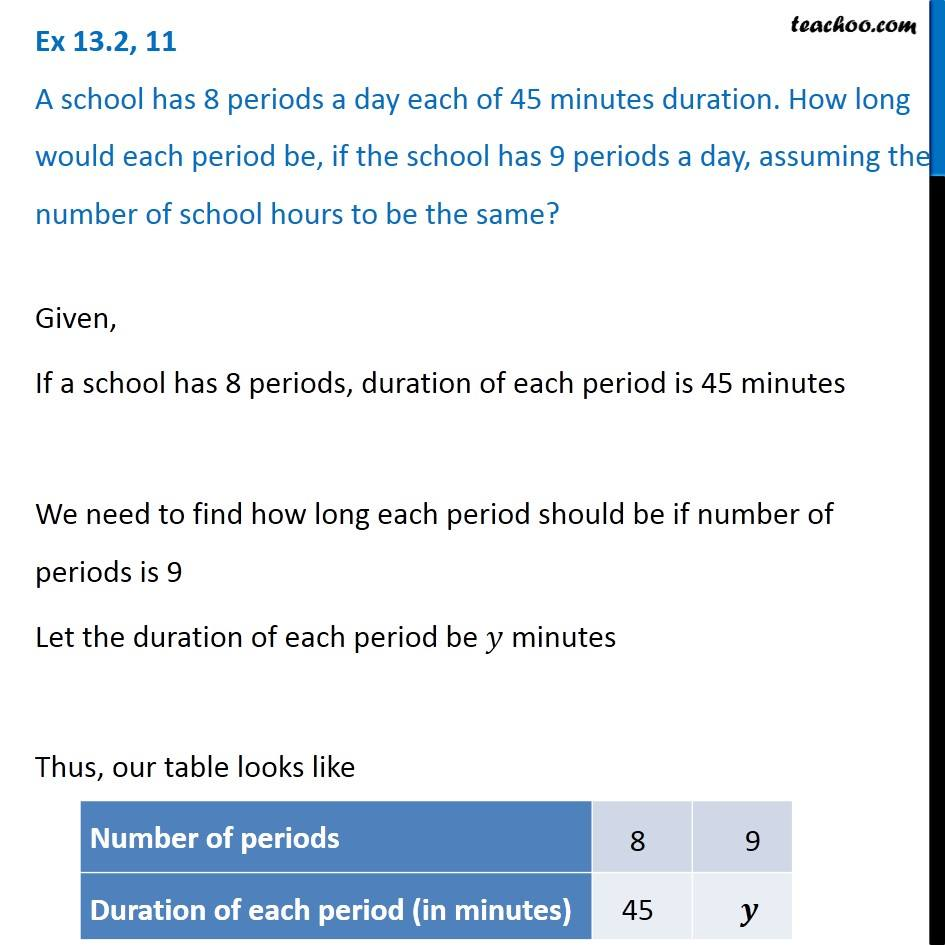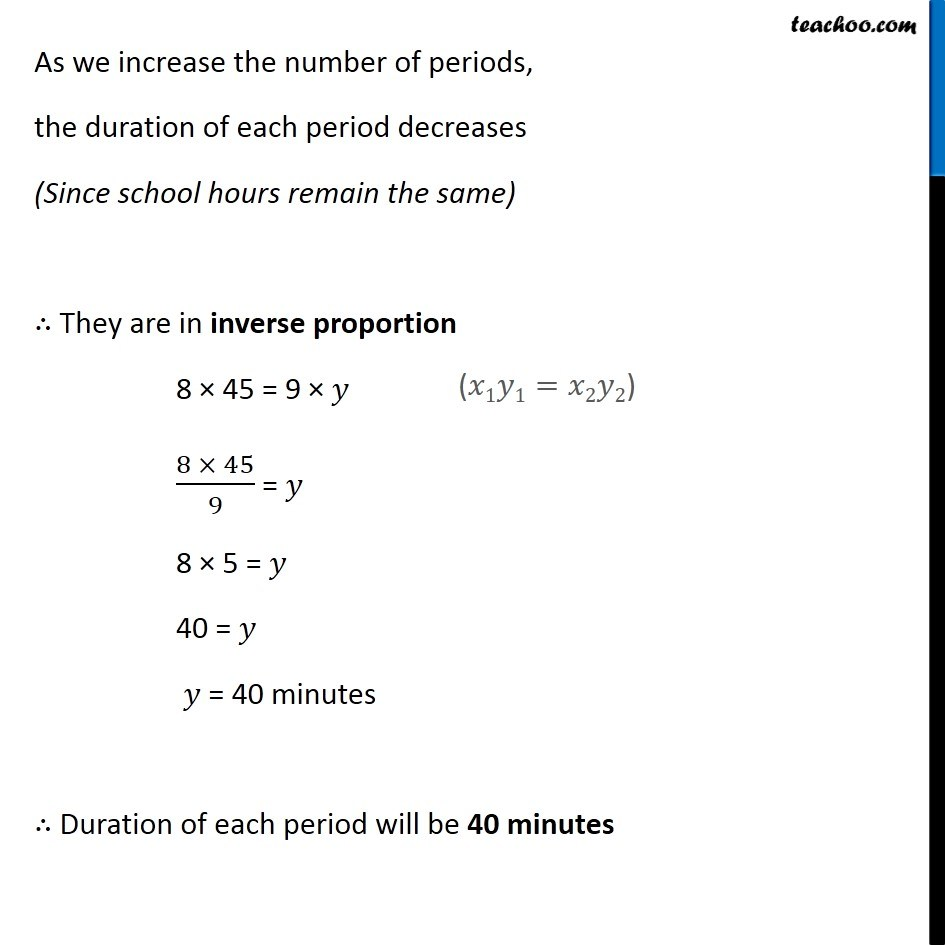Inverse Proportion

Chapter 13 Class 8 Direct and Inverse Proportions
Concept wise### Transcript

Ex 13.2, 11 A school has 8 periods a day each of 45 minutes duration. How long would each period be, if the school has 9 periods a day, assuming the number of school hours to be the same? Given, If a school has 8 periods, duration of each period is 45 minutes We need to find how long each period should be if number of periods is 9 Let the duration of each period be 𝑦 minutes Thus, our table looks like As we increase the number of periods, the duration of each period decreases (Since school hours remain the same) ∴ They are in inverse proportion 8 × 45 = 9 × 𝑦 (8 × 45)/9 = 𝑦 8 × 5 = 𝑦 40 = 𝑦 𝑦 = 40 minutes ∴ Duration of each period will be 40 minutes